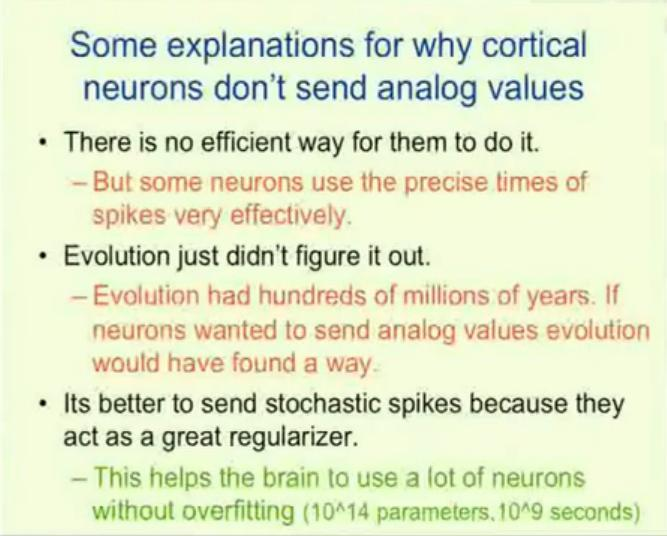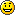# November 2012

You are currently browsing the monthly archive for November 2012.

## “Brains, Sex, and Machine Learning”

Hinton has a new Google tech talk “Brains, Sex, and Machine Learning“.  I think that if you are into neural nets, you’ve got to watch this video.  Here’s the abstract.

Recent advances in machine learning cast new light on two puzzling biological phenomena. Neurons can use the precise time of a spike to communicate a real value very accurately, but it appears that cortical neurons do not do this. Instead they send single, randomly timed spikes. This seems like a clumsy way to perform signal processing, but a recent advance in machine learning shows that sending stochastic spikes actually works better than sending precise real numbers for the kind of signal processing that the brain needs to do. A closely related advance in machine learning provides strong support for a recently proposed theory of the function of sexual reproduction. Sexual reproduction breaks up large sets of co-adapted genes and this seems like a bad way to improve fitness. However, it is a very good way to make organisms robust to changes in their environment because it forces important functions to be achieved redundantly by multiple small sets of genes and some of these sets may still work when the environment changes. For artificial neural networks, complex co-adaptations between learned feature detectors give good performance on training data but not on new test data. Complex co-adaptations can be reduced by randomly omitting each feature detector with a probability of a half for each training case. This random “dropout” makes the network perform worse on the training data but the number of errors on the test data is typically decreased by about 10%. Nitish Srivastava, Alex Krizhevsky, Ilya Sutskever and Ruslan Salakhutdinov have shown that this leads to large improvements in speech recognition and object recognition.

Hinton has a lot of great ideas in this video including this slide on a massively parallel approach to neural nets.And this oneAnd, as mentioned in the abstract, the idea of “dropouts” is very important. (Similar to denoising.)

I wonder if the idea of dropouts can be applied to create more robust Bayesian networks / Probabilistic Graphical Models.  Maybe the same effect can be achieved by introducing a bias (regularization) against connections between edges (similar to the idea of sparsity).

## Matroids

Matroids are an abstraction of Vector Spaces.  Vectors spaces have addition and scalar multiplication and the axioms of vectors spaces lead to the concepts of subspaces, rank, and independent sets.  All Vector Spaces are Matroids, but Matroids only retain the properties of independence, rank, and subspaces. The idea of the Matroid closure of a set is the same as the span of the set in the Vector Space.

## 100 Most useful Theorems and Ideas in Mathematics

So I have been thinking about which ideas in mathematics I use most often and I have listed them below.  Please feel free to comment because I would like to use this list as a starting point for a future list of “The most useful ideas in Mathematics for Scientists and Engineers”.
$\$
I showed this list to Carl and he said his list would be completely different.  (He choked a bit when he saw primes at #71.)  Hopefully we can post his list as well as others.
$\$
1. counting
2. zero
3. integer decimal positional notation 100, 1000, …
4. the four arithmetic operations + – * /
5. fractions
6. decimal notation  0.1, 0.01, …
7. basic propositional logic (Modus ponens, contrapositive, If-then, and, or, nand, …)
8. negative numbers
9. equivalence classes
10. equality & substitution
11. basic algebra – idea of variables, equations, …
12. the idea of probability
13. commutative and associative properties
14. distributive property
15. powers (squared, cubed,…),  – compound interest (miracle of)
16. scientific notation 1.3e6 = 1,300,000
17. polynomials
18. first order predicate logic
19. infinity
20. irrational numbers
21. De Morgan’s laws
22. statistical independence
23. the notion of a function
24. square root  (cube root, …)
25. inequalities (list of inequalities)
26. power laws (i.e. $a^b a^c = a^{b+c}$ )
27. Cartesian coordinate plane
28. basic set theory
29. random variable
30. probability distribution
31. histogram
32. the meanexpected value & strong law of large numbers
33. the graph of a function
34. standard deviation
35. Pythagorean theorem
36. vectors and vector spaces
37. limits
38. real numbers as limits of fractions, the least upper bound
39. continuity
40. $R^n$, Euclidean Space,  and Hilbert spaces (inner or dot product)
41. derivative
42. correlation
43. central limit theorem, Gaussian Distribution, Properties of Guassains.
44. integrals
45. chain rule
46. modular arithmetic
47. sine cosine tangent
48. $\pi$circumference, area, and volume formulas for circles, rectangles, parallelograms, triangles, spheres, cones,…
49. linear regression
50. Taylor’s theorem
51. the number e and the exponential function
52. Rolle’s theoremKarush–Kuhn–Tucker conditions, derivative is zero at the maximum
53. the notion of linearity
54. Big O notation
55. injective (one-to-one) / surjective (onto) functions
56. imaginary numbers
57. symmetry
58. Euler’s Formula $e^{i \pi} + 1 = 0$
59. Fourier transform, convolution in time domain is the product in the frequency domain (& vice versa), the FFT
60. fundamental theorem of calculus
61. logarithms
62. matrices
63. conic sections
64. Boolean algebra
65. Cauchy–Schwarz inequality
66. binomial theorem – Pascal’s triangle
67. the determinant
68. ordinary differential equation (ODE)
69. mode (maximum likelihood estimator)
70. cosine law
71. prime numbers
72. linear independence
73. Jacobian
74. fundamental theorem of arithmetic
75. duality – (polyhedron faces & pointsgeometry lines and pointsDual Linear Programdual space, …)
76. intermediate value theorem
77. eigenvalues
78. median
79. entropy
80. KL distance
81. binomial distribution
82. Bayes’ theorem
83. $2^{10} \approx 1000$
84. compactnessHeine – Borel theorem
85. metric space, Triangle Inequality
86. ProjectionsBest Approximation
87. $1/(1-X) = 1 + X + X^2 + \ldots$
88. partial differential equations
90. Reisz representation theorem
91. Fubini’s theorem
92. the ideas of groups, semigroups, monoids, rings, …
93. Singular Value Decomposition
94. numeric integration – trapezoidal rule, Simpson’s rule, …
95. mutual information
96. Plancherel’s theorem
97. matrix condition number
98. integration by parts
99. Euler’s method for numerical integration of ODEs (and improved EulerRunge–Kutta)
100. pigeon hole principle

## History of Compressed Sensing

Igor Carron provides a nice history of Compressed Sensing on his blog.  He reviews the computation complexity of various Compressed Sensing algorithms, noting that the field started with the discovery of polynomial time solutions to underdetermined linear problems.  While $l_0$ norm regularization results in NP-complete problems, $l_1$ regularization is easier.

## TIL: A trick for hits on your blog

If you want hits on your blog, write about an article that is being read by thousands or millions of people.  Some of those readers will Google terms from the article. Today I blogged very briefly about the NY Times article “Scientists See Promise in Deep-Learning Programs” and I was surprised at the number of referrals from Google.  Hmm, maybe I should blog about current TV shows (Big Bang? Mythbusters?), movies (Cloud Atlas?), and video games (Call of Duty?).   Carl suggested that I apply deep learning, support vector machines, and logistic regression to estimate the number of hits I will get on a post.  If I used restricted Boltzmann machines, I could run it in generative mode to create articlesI was afraid if I went down that route I would eventually have a fantastically popular blog about Britney Spears.

## Deep Learning in The NY Times

John Markoff at the NY times writes about recent advances in and implications of deep neural nets in “Scientists See Promise in Deep-Learning Programs“.  He reviews the recent activities of  Hinton, LeCun, and Ng as well as applications of deep learning in speech recognition, computer vision, chemistry (Kaggel Merck Prize), and near real-time natural language processing and machine translation.

## Gauss’s continued fraction

Gauss did a lot of math, but sometimes I am surprised when I find something new to me done by Gauss

$\tan(x) = { 1 \over{1/x \ – {1\over{3/x \ – {1\over{5/x\ – \ldots}}}}}}$

## “Clustering via Dirichlet Process Mixture Models for Portable Skill Discovery”

In “Clustering via Dirichlet Process Mixture Models for Portable Skill Discovery“, Niekum and Barto (2011) apply Sub-goal Discovery and Skill Discovery to improve Reinforcement Learning on Markov Decision Processes.  They write,

“Skill discovery algorithms in reinforcement learning typically identify single states or regions in state space that correspond to task-speciﬁc subgoals. However, such methods do not directly address the question of how many distinct skills are appropriate for solving the tasks that the agent faces. This can be highly inefficient when many identified subgoals correspond to the same underlying skill, but are all used individually as skill goals. For example, opening a door ought to be the same skill whether an agent is one inch or two inches away from the door, or whether the door is red or blue; making each possible configuration a separate skill would be unwise. Furthermore, skills created in this manner are often only transferable to tasks that share identical state spaces, since corresponding subgoals across tasks are not merged into a single skill goal.

We show that these problems can be overcome by collecting subgoal data from a series of tasks and clustering it in an agent-space , a shared feature space across multiple tasks. The resulting clusters generalize subgoals within and across tasks and can be used as templates for portable skill termination conditions. Clustering also allows the creation of skill termination conditions in a datadriven way that makes minimal assumptions and can be tailored to the domain through a careful choice of clustering algorithm. Additionally, this framework extends the utility of single-state subgoal discovery algorithms to continuous domains, in which the agent may never see the same state twice. We argue that clustering based on a Dirichlet process mixture model is appropriate in the general case when little is known about the nature or number of skills needed in a domain. Experiments in a continuous domain demonstrate the utility of this approach and illustrate how it may be useful even when traditional subgoal discovery methods are infeasible.”

They apply Dirichlet process mixture models (more specifically an inﬁnite Gaussian mixture model with a Dirichlet prior on the number of Gaussians and the parameters of the Gaussians) using expectation-maximization to do clustering of the states—each cluster corresponding to a stratagem referred to as a “latent option”.  Experimental results in an environment similar to the Lightworld domain (see “Building Portable Options: Skill Transfer in Reinforcement Learning” by Konidaris and  Barto (2007)) are reported.

## Topological Sorting

Given a directed a-cyclic graph (DAG) find an ordering of the nodes which is consistent with the directed edges.  This is called Topological Sorting and several algorithms exist.

## New Developments in Iterated Prisoner’s Dilemma

In “Iterated Prisoner’s Dilemma contains strategies that dominate any evolutionary opponent” by Pressa and Dyson (2012) show that there are strategies, named “zero determinant strategies” (ZD) which “win” against so-called evolutionary strategies in Prisoner’s Dilemma.  An easy to read, less mathematical summary of the paper is given in “Extortion and cooperation in the Prisoner’s Dilemma” by Stewart  and Plotkin.  Fans of Tit-for-Tat need not fear because Tit-for-Tat does not lose significantly against the ZD strategies. The idea of the ZD strategies is not very complicated.   The ZD player cooperates enough that it is profitable to cooperate with the ZD player, but the ZD player defects enough that 1) it is not profitable to defect against the ZD player, 2) the ZD player wins more than his opponent when the opponent cooperates.

« Older entries